PRAVEEN KUMAR

•Activity

PRAVEEN KUMAR

•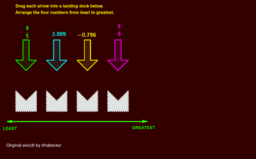TG Ordering Rational Numbers

Activity

PRAVEEN KUMAR

•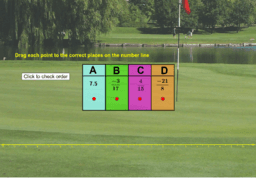TG Ordering Rational Numbers 1

Activity

PRAVEEN KUMAR

•TG Ordering Rational Numbers

Activity

PRAVEEN KUMAR

•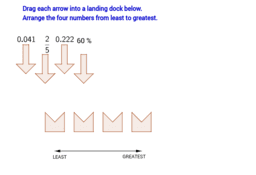TG Arrange fractions, decimals, and percents

Activity

PRAVEEN KUMAR

•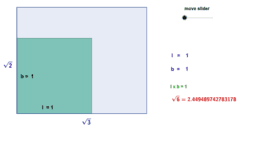TG Geometrical Meaning of product of irrational numbers

Activity

PRAVEEN KUMAR

•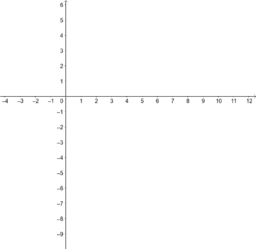TG Interesting patterns in rational numbers from MathsJam 2015

Activity

PRAVEEN KUMAR

•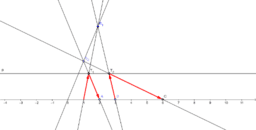TG ProjectiveMultiplication

Activity

PRAVEEN KUMAR

•TG fraction operations

Activity

PRAVEEN KUMAR

•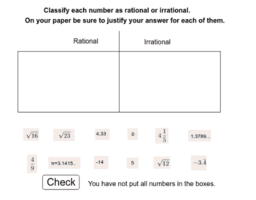TG RationalSort

Activity

PRAVEEN KUMAR

•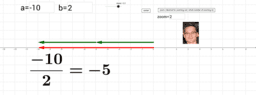TG division of signed numbers

Activity

PRAVEEN KUMAR

•Activity

PRAVEEN KUMAR

•TG Whole number

Activity

PRAVEEN KUMAR

•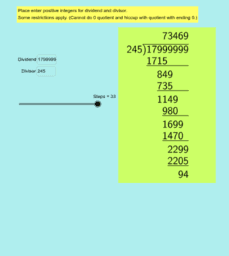TG Long Division with Whole Numbers

Activity

PRAVEEN KUMAR

•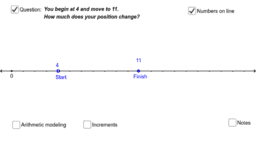TG ChangeWholeNumbers

Activity

PRAVEEN KUMAR

•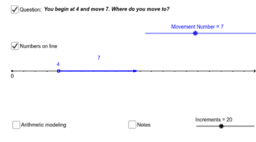TG MoveToWholeNumbers

Activity

PRAVEEN KUMAR

•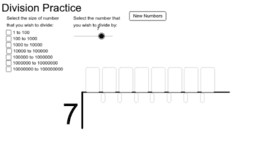TG Practice with division (whole numbers)

Activity

PRAVEEN KUMAR

•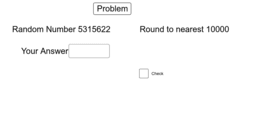TG Rounding Whole Numbers

Activity

PRAVEEN KUMAR

•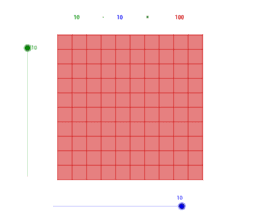TBG Multiplication of Natural Numbers

Activity

PRAVEEN KUMAR

•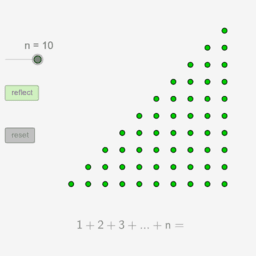TG The Sum of the First n Natural Numbers - Dynamic Proof

Activity

PRAVEEN KUMAR

•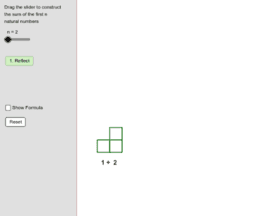TG The Sum of the First n Natural Numbers - Dynamic Geometric P

Activity

PRAVEEN KUMAR

•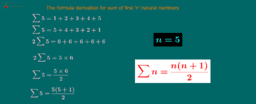TG Sum of first 'n' natural numbers

Activity

PRAVEEN KUMAR

•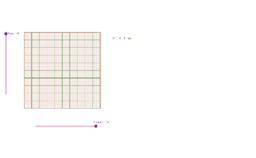TG Visualizing Multiplication of Natural Numbers

Activity

PRAVEEN KUMAR

•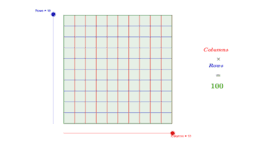TG Multiplication of Natural Numbers

Activity

PRAVEEN KUMAR